The Heptagons ClipArt gallery contains 15 illustrations of closed two-dimensional geometric figures with seven sides. Images include examples of regular (those having 7 equal sides and 7 equal angles), irregular, concentric, concave, and convex heptagons. Heptagons are also known as septagons.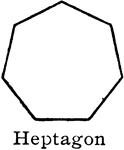### Heptagon

"A polygon of...seven sides [is] a heptagon." —Hallock 1905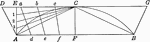### Construction of Regular Heptagon in a Circle

Illustration of beginning of construction of a regular heptagon in a circle.### Irregular heptagon

Illustration of an irregular polygon/heptagon/septagon.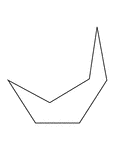### Irregular Concave Heptagon

Illustration of an irregular concave heptagon.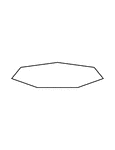### Irregular Convex Heptagon

Illustration of an irregular convex heptagon. This polygon has some symmetry.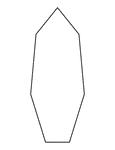### Irregular Convex Heptagon

Illustration of an irregular convex heptagon. This polygon has some symmetry.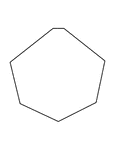### Irregular Convex Heptagon

Illustration of an irregular convex heptagon. This polygon has some symmetry.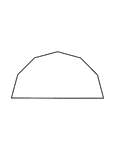### Irregular Convex Heptagon

Illustration of an irregular convex heptagon. This polygon has some symmetry.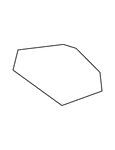### Irregular Convex Heptagon

Illustration of an irregular convex heptagon.### 7-sided Polygon

Polygon consisting of 7 sides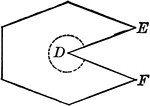### Concave Polygon

Illustration of a concave polygon. A concave polygon is a polygon of which tow or more sides, if produced,…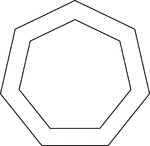### 2 Concentric Septagons/Heptagons

Illustration of 2 regular concentric heptagons/septagons.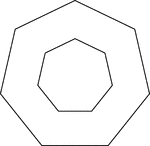### 2 Concentric Septagons/Heptagons

Illustration of 2 regular concentric heptagons/septagons.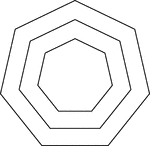### 3 Concentric Septagons/Heptagons

Illustration of 3 regular concentric heptagons/septagons that are equally spaced.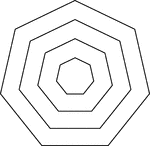### 4 Concentric Septagons/Heptagons

Illustration of 4 regular concentric heptagons/septagons that are equally spaced.